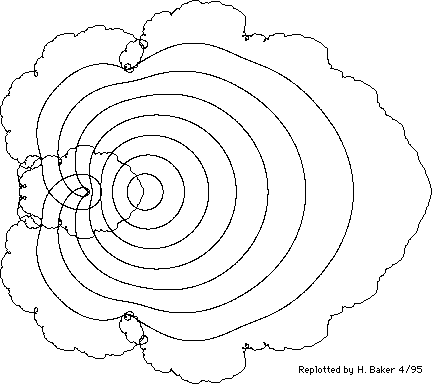Up

Figure 6(b). [Item 123] Image of circles |z| = 1/8, 2/8, ..., 8/8 under the function

```                      inf     n
===    2
\     z
f(z) =  >    --- .
/      n
===   2
n=0
```
Both [original] plots by Salamin on the RLE PDP-1.Figure 6(b). [Item 123] Image of circles |z| = 1/8, 2/8, ..., 8/8 under the function

```                      inf     n
===    2
\     z
f(z) =  >    --- .
/      n
===   2
n=0
```
Both [original] plots by Salamin on the RLE PDP-1.

[Retyped and formatted in html ('Web browser format) by Henry Baker, April, 1995.]